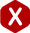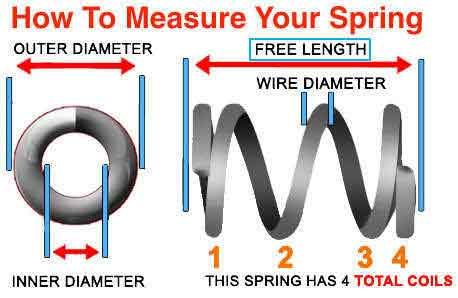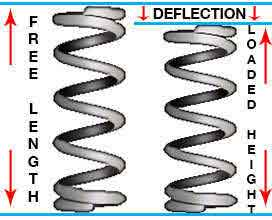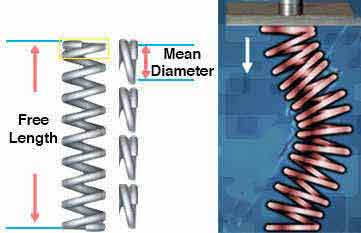<# Free Length of Spring

#### Definition: The actual length of a compression spring without any sort of load or force being placed on it.

When a load or force is placed on a compression spring, its length deflects. Even if the load or force is very small, the length of the spring may travel a few thousandths of an inch. The amount of travel it will deflect depends on the spring’s strength/stiffness and the amount of load being placed. The free length of your spring is the length it has right when it comes off the machine. It is the length without those loads or forces.

If a compression spring’s free length is too long in proportion to its diameter, it is going to bend/buckle. This is referred to as the Slenderness Ratio. In order to calculate your spring’s slenderness ratio, you must calculate the mean diameter first by subtracting the wire diameter from the outer diameter. The mean diameter is the diameter right in between the outer diameter and the inner diameter; measured from center wire to center wire. Then, you must divide the free length of spring by the mean diameter. The result of this equation will be written as x:1 (x to 1) describing the proportion being made to 1 mean diameter as shown in the example to the right.

The free length of your spring, paired with other dimensions, may also be elongated to provide you with more travel thus requiring more force. This method is suggested when you need a stronger spring but are under very tight tolerances thus making it difficult to change any other dimension. To know if you’re able to do this with the free length of your spring, visit our online spring calculator and run a design. Look for the values True Maximum Travel and Maximum Travel Considering Solid Height. The maximum travel considering solid height is the amount of travel you’re able to achieve out of your current design with the current free length of spring. The True Maximum Travel, on the other hand, is the travel you would be able to get out of your spring if you made the free length a bit longer. If these two values are the same, you will not be able to make your spring’s free length any longer, but if the True Maximum Travel is greater than the Maximum Travel Considering Solid height, you are able to extend it.

To calculate how much you are able to extend your compression spring’s free length, you must subtract the Maximum Travel Considering Solid Height from the True Max Travel. Once you’ve done this, you’re able to add a value less than or equal to (≤) the result you got from the previous calculation.